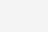## 基本概念1. 1. 从教练参加大赛代表角度看，这两名选手得分一样，但是左下选手成绩较为稳定，以较为稳定的心理素质顶住了比赛的压力，稳定发挥着自己的真实水平，这在大赛中不会有大的起伏，必然值的信任。
2. 2. 从教练选才的角度看，相同成绩的结果，但是右上选手可以打出中心成绩，说明有较高的潜力可以开发；而与此同时左下选手则较为平庸，虽然心理素质稳定，也恰恰说明该选手的极限能力就在于此了，难以开发。所以，右上选手更值得培养。

## 偏差方差分解

$$MSE=\frac{1}{m}\sum_{i=1}^{m}(y_i-\hat h(x_i))^2=E[(y-\hat h(x))^2]$$

$$\begin{equation} \begin{split} E[(y-\hat h(x))^2] & = E[y^2+\hat h(x)^2-2y*\hat h(x)] \\ & = E[y^2]+E[\hat h(x)^2]-2E[y*\hat h(x)] \\ & = Var[y]+E[y^2]+Var[\hat h(x)]+(E[\hat h(x)])^2-2*E[\hat h(x)]E[y] \\ & = Var[y]+Var[\hat h(x)]+(h(x)-E[\hat h(x)])^2 \\ & = sigma^2+Var[\hat h(x)]+Bias(\hat h(x)) \end{split} \end{equation}$$

$$Var[y]=E[y^2]-E[y]^2 导出 E[y^2]=Var[y]+E[y]^2$$

$$y=h(x)+\epsilon 导出 E[y]=E[h(x)+\epsilon]=E[h(x)]+E[\epsilon]=h(x)$$

$$Var[y]=E[(y-E[y])^2]=E[(y-(y-\epsilon))^2]=E[\epsilon^2]=Var[\epsilon]+E[\epsilon]=\sigma^2$$

1. 1. $$\sigma^2$$是数据的固有噪声，与模型训练系统无关，无法改变。
2. 2. $$Var[\hat h(x)]$$是训练模型的方差，为了最小化MES，也就要求模型稳定，实质就是多次训练变化较小。
3. 3. $$Bias(\hat h(x))$$是训练模型的偏差，为了最小化MSE,就要求模型最大限度的拟合真实函数。

## 实验分析

1. 1. 数据通过对$$sin(2\pi *x)$$随机采样100点，同时添加均值为0，方差为1的高斯白噪声。同时生成这样的数据20组。
2. 2. 采用多项式拟合数据，依次采用3阶多项式、9阶多项式、15阶多项式对数据进行拟合。## 偏差方差应用

#### 最小化偏差

1. 1. 增加参数，通过增加可以调节的参数数量，达到提升模型复杂度的目的。借助复杂的模型规则更好的拟合真实函数，进而减小方差。在本文实验中通过增加幂指数项个数的方法就是实现这个目的。
2. 2. Boosting,就是通过多个弱分类器组合成强分类器的思想。而这里的弱分类器往往就是较为简单的模型，它是通过多个弱分类器对数据进行分析，经过投票，通过综合获得最终的分类结果。
3. 3. …

#### 正则化

1. 1. 熟悉模式识别的人都熟悉模型训练中过于复杂的模型容易造成过拟合，而解决过拟合的首选方法就是正则化，通过正则化来限制模型有效参数的个数，达到降低模型复杂度的目的，进而实现降低方差的目标。
2. 2. Bagging,该方法通过给定的算法，对原始数据集进行随机采样，并构建模型。最后通过多个模型的综合，作为最终的模型输出。提高了结果的稳定性，降低了训练模型的方差。
3. 3. …